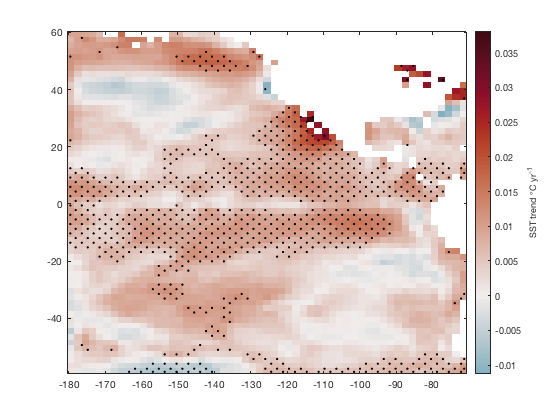# stipple documentation

stipple creates a hatch filling or stippling within a grid. This function is designed primarily to show regions of statistical significance in spatial maps.

## Syntax

```stipple(x,y,mask)
stipple(...,MarkerProperty,MarkerValue,...)
stipple(...,'density',DensityValue)
stipple(...,'resize',false)
h = stipple(...)```

## Description

stipple(x,y,mask) plots black dots in x,y locations wherever a mask contains true values. Dimensions of x, y, and mask must all match.

stipple(...,MarkerProperty,MarkerValue,...) specifies any marker properties that are accepted by the plot function (e.g., 'color', 'marker', 'markersize', etc).

stipple(...,'density',DensityValue) specifies density of stippling markers. Default density is 100, but if your plot is too crowded you may specify a lower density value (and/or adjust the markersize).

stipple(...,'resize',false) overrides the 'density' option and plots stippling at the exact resolution of the input grid. By default, the grids are resized because any grid larger than about 100x100 would produce so many stippling dots it would black out anything under them.

h = stipple(...) returns a handle of the plotted stippling objects.

## Example 1

Here's an example you can try at home, which uses peaks to create a 1000x1000 grid of data:

```% Load some sample data:
[X,Y,Z] = peaks(1000);

pcolor(X,Y,Z)
hold on
```Let's say everywhere Z exceeds 2.5 should have stippling:

```mask = Z>2.5;
```## Example 2: Specify color

If you prefer gray dots to the default black dots, do this:

```stipple(X,Y,mask,'color',0.5*[1 1 1])
```## Example 3: Specify density

Too many dots? Specify whatever density you'd like. The default density is 100, so values lower than that will produce fewer dots, whereas higher values produce more dots. (Specify 'resize',false if you want the density to exactly match the input grids.).

```figure
pcolor(X,Y,Z)
hold on
```## Example 4: Specify several options at once:

To set everything just the way you like it, specify as many options as you'd like. Here we'll plot big red plus signs:

```stipple(X,Y,mask,'density',75,'color','r','marker','+','markersize',9)
```## Example 5: Regions of statistical significance

Here's a real-world application of stippling: Denote regions of statistically significant sea surface temperature trends with stipples. First, load the sample pacific_sst.mat dataset, which contains monthly gridded sea surface temperature data, and calculate trends and corresponding p-values with trend:

```load pacific_sst

[tr,p] = trend(sst,12);

figure
imagescn(lon,lat,tr)
cb = colorbar;
ylabel(cb,'SST trend \circC yr^{-1}')
cmocean('balance','pivot') % sets the colormap with zero in the middle
```Define statistical significance by, let's say, anything with a p-value of less than 0.01:

```StatisticallySignificant = p<0.01;
```

Now plot with stipple:

```hold on
stipple(lon,lat,StatisticallySignificant)
```## Author Info

This function was written by Chad A. Greene, August 2018.